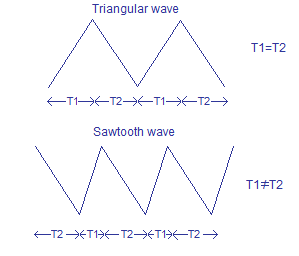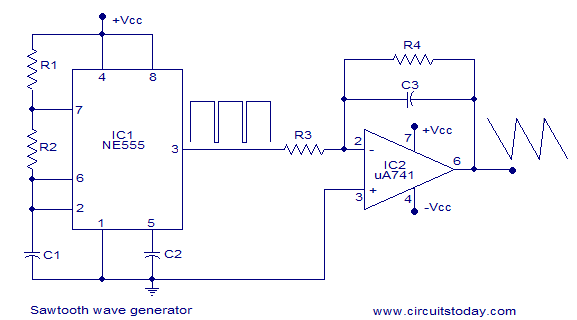## Audio

[Audio][slideshow]

### Sawtooth wave generator.

Many people often get confused between sawtooth wave and a triangular wave. Sawtooth waveform is a type of linear non sinusoidal waveform with a triangular shape in which the rise time and fall time are different. A pure triangular waveform is is also linear, non sinusoidal and have a triangular shape but it has equal rise and fall times.The sawtooth waveform can also be called an assymmetric triangular wave. The graphical representation of a triangular and sawtooth waveform are shown in the figure below. T1 is assumed to be the rise time and T2 is assumed to be the fall time.Like triangular waveforms, sawtooth waveforms are also used in applications like thyristor (SCR, triac etc) switching, sampling, tone/frequency generation, modulation etc.

#### Sawtooth wave generator using NE555 timer and uA741 opamp.

Sawtooth waveforms can be generated by integrating square waves with unequal rise and fall times (asymmetrical square waves). The circuit shown below shows a setup for generating sawtooth wave. Here an NE555 timer IC is used for generating the assymmetric square wave and opamp integrator based on uA741 is used for integrating the assymmetric square wave. The circuit diagram is shown below.Here the IC NE555 is wired as an astable multivibrator with unequal ON and OFF times. The the resistor R1, R2 and capacitor C2 sets the ON and OFF time periods. The value of these components are so selected that the OFF time is less than 10% of the ON times. The asymmetric square wave is available at pin 3 of the IC. The working of the astable multivibrator is given below.

When the power supply is switched ON, capacitor C1 starts charging through resistors R1 and R2. When the voltage across C1 is above 2/3Vcc the upper comparator inside the NE555 swings to positive saturation and this triggers the internal flip-flop. This makes the output (pin 3) of the timer low. Now the capacitor C1 starts to discharge through resistor R2 into pin 7 of the IC. When the voltage across capacitor C1 becomes less than 1/3Vcc, the lower comparator inside the IC switches to positive saturation and this again triggers the internal flip-flop. As a result the output of the timer( pin 3) goes low. This action is repeated and the result will be a square wave at pin 3 of the NE555. The charging time period (ON time) is given by the equation T1= 0.69(R1+R2)C1 and the discharging time period (OFF time) is given by the equation T2=0.69R2C1. Read this article : NE555 as astable multivibrator for a better understanding on NE555 based astable multivibrators..

The assymmetric square wave obtained at the output of NE555 is integrated by the inverting active integrator based on opamp IC uA741. Resistors R3 and R4 sets the gain of the opamp integrator. Resistor R4 in conjunction with capacitor C3 sets the bandwidth. Since the integrator is wired in inverting mode, the sawtooth wavform falls when the timer output is high and rises when the timer output is low. Read this article: Triangular wave generator for a better understanding on opamp integrators.

#### Testing the sawtooth wave generator.

Practical circuit diagram, design and testing videos+photographs of the sawtooth wave generator will be added soon.

[Lights][grids]